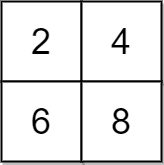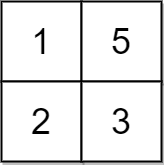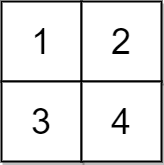##### Welcome to Subscribe On Youtube

Formatted question description: https://leetcode.ca/all/2033.html

# 2033. Minimum Operations to Make a Uni-Value Grid (Medium)

You are given a 2D integer grid of size m x n and an integer x. In one operation, you can add x to or subtract x from any element in the grid.

A uni-value grid is a grid where all the elements of it are equal.

Return the minimum number of operations to make the grid uni-value. If it is not possible, return -1.

Example 1:Input: grid = [[2,4],[6,8]], x = 2
Output: 4
Explanation: We can make every element equal to 4 by doing the following:
- Add x to 2 once.
- Subtract x from 6 once.
- Subtract x from 8 twice.
A total of 4 operations were used.


Example 2:Input: grid = [[1,5],[2,3]], x = 1
Output: 5
Explanation: We can make every element equal to 3.


Example 3:Input: grid = [[1,2],[3,4]], x = 2
Output: -1
Explanation: It is impossible to make every element equal.


Constraints:

• m == grid.length
• n == grid[i].length
• 1 <= m, n <= 105
• 1 <= m * n <= 105
• 1 <= x, grid[i][j] <= 104

Similar Questions:

## Solution 1. Left-to-right State Transition

// OJ: https://leetcode.com/problems/minimum-operations-to-make-a-uni-value-grid/
// Time: O(NlogN) where N is the count of all elements in A.
// Space: O(N)
class Solution {
public:
int minOperations(vector<vector<int>>& A, int x) {
vector<int> B;
for (auto &r : A) {
for (int n : r) B.push_back(n);
}
sort(begin(B), end(B));
long mn = B, val = 0;
for (int i = 0; i < B.size(); ++i) {
if ((B[i] - mn) % x) return -1;
val += (B[i] - mn) / x;
}
long ans = val;
for (int i = 1; i < B.size(); ++i) {
int diff = (B[i] - B[i - 1]) / x;
val += i * diff - (B.size() - i) * diff;
ans = min(ans, val);
}
return ans;
}
};


## Solution 2. Median Minimizes Sum of Absolute Deviations

Any median minimizes the sum of absolute deviations. (Reference)

If the array has odd number of elements, there is only a single median which minimizes the sum of absolute deviations.

If the array has even number of elements, any numbers between (including) the two median numbers minimizes the sum of absolute deviations.

// OJ: https://leetcode.com/problems/minimum-operations-to-make-a-uni-value-grid/
// Time: O(NlogN) where N is the count of all elements in A.
// Space: O(N)
class Solution {
public:
int minOperations(vector<vector<int>>& A, int x) {
vector<int> B;
for (auto &r : A) {
for (int n : r) B.push_back(n);
}
sort(begin(B), end(B));
int median = B[B.size() / 2], ans = 0;
for (int i = 0; i < B.size(); ++i) {
int d = abs(B[i] - median);
if (d % x) return -1;
ans += abs(d) / x;
}
return ans;
}
};